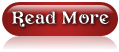## EMI Calculator for Home Loan, Car Loan & Personal Loan in India

EMI stands for equated monthly installment, which is a fixed payment amount made by a borrower to a lender/bank at a specified date each calendar month.

EMIs(Equated monthly installments) are used to pay off both interest and principal each month. Please find below on line EMI calculator tool and below the actual formula which will be used to calculate the EMI amount, all banks will use the same formula to calculate the loan EMI amount.

To calculate the EMI amount, please enter your principal amount, rate of interest and the duration in months then click the calculate button.

 Loan Amount (Principal): Rs. Interest Rate: % Tenor (No of Months): Months. Equated Monthly Installments (EMI): Rs. Total Amount with Interest: Rs. Total Interest Amount: Rs.

Note:
• Calculator is provided only as general self-help Planning Tools.
• Calculated EMI Result is indicative only.

Formula

EMI =P × R × (1 + R)n/((1 + R)n - 1)

P= principal (amount of loan),
R = rate of interest per installment period, i.e., if interest is 12% p.a. R = 1,
n = no. of installments in the tenure,
^ denotes whole to the power.

Example 1:
Calculate the EMI for the loan amount taken Rs.20,00,000 (20 lakh) at 9% annual interest [(9/12)/100 = 0.75 per month/100] for a period of 15 years (180 months).
Here P = 20,00,000 and R = 0.75/100 (9%/12) and n = 180 (15 years)
EMI = P × R × [ (1 + R)n/((1 + R)n - 1) ]
EMI = (20,00,000 x 0.75/100) x [ (1+0.75/100)180/ ((1+0.75/100)180-1) ]
= (20,00,000 x 0.0075) x [ (1+0.0075)180 / ((1+0.0075)180-1) ]
= 15,000 x [ (1.0075)180 / ((1.0075)180-1) ]
= 15,000 x [ (3.8380432675) / (3.8380432675)-1) ]
= 15,000 x [ (3.8380432675) / (2.8380432675) ]
= 15,000 x [ (1.352355445546427) ]
= Rs 20,285

Example 2:
Ravi has borrowed Rs. 5 lakhs from a bank on the interest rate of 12% (annual interest) for 5 years. Here P = 5,00,000 and R = (12/12)/100 = 1 per month/100 = 0.01 and n = 60 (5 years)
Loan means lending money from one individual or entity to another. A loan has three components – principal or the borrowed amount, rate of interest and tenure or duration for which the loan is availed.